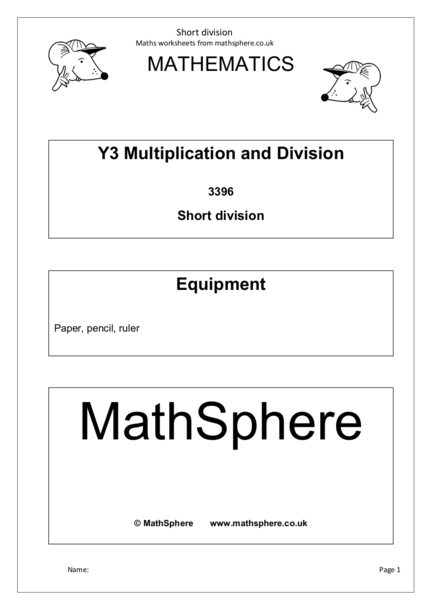# Short Division Method: ExplainedContributed by:Short Division Method. In arithmetic, the short division is a division algorithm that breaks down a division problem into a series of easier steps. It is an abbreviated form of long division — whereby the products are omitted and the partial remainders are notated as superscripts.
1. Short division
Maths worksheets from mathsphere.co.uk
MATHEMATICS
Y3 Multiplication and Division
3396
Short division
Equipment
Paper, pencil, ruler
MathSphere
Name: Page 1
2. Short division
Maths worksheets from mathsphere.co.uk
Today we are going to look at how we can use the
short method of division. Short, because it uses up
less space! Try it, step by step.
Divide 3 into 56, one digit at a time,
starting from the left like this:
3)5 6
Ask: ‘How many 3s in 5?’
3 goes into 5 1 time with 2 remainder.
Put the 1 above the 5 on the answer line. 1
3 ) 526
Carry the 2 into the next column and write it
down before the 6, making 26.
Ask: ‘How many 3s in 26?’
3 goes into 26 8 times. 18
3 ) 526
Put the 8 above the 6 on the answer line.
3 times 8 is 24 so there is a remainder of 2.
Put the 2 remainder in the answer. 18r2
Answer 18 remainder 2. 3 ) 526
Name: Page 2
3. Short division
Maths worksheets from mathsphere.co.uk
Use the short method of division to
answer these questions. Some will have
remainders.
1. 2) 5 2 2. 2) 4 8 3. 2) 7 5 4. 2) 3 7
5. 3) 5 3 6. 3) 7 1 7. 3) 8 6 8. 3) 7 4
9. 4) 5 3 10. 4) 6 5 11. 4) 7 7 12. 4) 5 9
13. 5) 7 2 14. 5) 3 9 15. 5) 4 8 16. 5) 9 7
Remember to include any
Name: Page 3
4. Short division
Maths worksheets from mathsphere.co.uk
Use the short method of division to
answer these questions. Some will have
remainders.
1. 2) 3 5 2. 2) 5 9 3. 2) 8 6 4. 2) 4 9
5. 3) 7 8 6. 3) 8 2 7. 3) 9 7 8. 3) 6 4
9. 4) 5 6 10. 4) 7 0 11. 4) 9 3 12. 4) 3 9
13. 5) 8 3 14. 5) 4 9 15. 5) 7 7 16. 5) 9 4
Remember to include any
Name: Page 4
5. Short division
Maths worksheets from mathsphere.co.uk
Use the short method of division to
answer these questions. Some will have
remainders.
1. 2) 7 0 2. 2) 6 6 3. 2) 9 3 4. 2) 5 5
5. 3) 7 2 6. 3) 9 1 7. 3) 5 4 8. 3) 8 5
9. 4) 7 1 10. 4) 8 6 11. 4) 9 5 12. 4) 8 3
13. 5) 9 1 14. 5) 5 7 15. 5) 6 6 16. 5) 3 7
Remember to include any
Name: Page 5
6. Short division
Maths worksheets from mathsphere.co.uk
Page 3
1. 26 2. 24 3. 37 r 1 4. 18 r 1
5. 17 r 2 6. 23 r 2 7. 28 r 2 8. 24 r 2
9. 13 r 1 10. 16 r 1 11. 19 r 1 12. 14 r 3
13. 14 r 2 14. 7 r 4 15. 9 r 3 16. 19 r 2
Page 4
1. 17 r 1 2. 29 r 1 3. 43 4. 24 r 1
5. 26 6. 27 r 1 7. 32 r 1 8. 28 r 1
9. 14 10. 17 r 2 11. 23 r 1 12. 9 r 3
13. 16 r 3 14. 9 r 4 15. 15 r 2 16. 18 r 4
Page 5
1. 35 2. 33 3. 46 r 1 4. 27 r 1
5. 24 6. 30 r 1 7. 18 8. 28 r 1
9. 17 r 3 10. 21 r 2 11. 23 r 3 12. 20 r 3
13. 18 r 1 14. 11 r 2 15. 13 r 1 16. 7 r 2
Name: Page 6